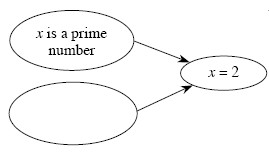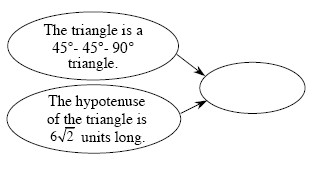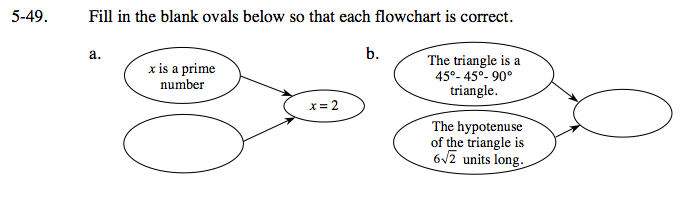### Home > GC > Chapter 5 > Lesson 5.2.1 > Problem5-49

5-49.
1. Fill in the blank ovals below so that each flowchart is correct. Homework Help ✎

1.2.The fact is x is a prime number so the conclusion is x = 2, so x = 2 is a prime number.
What is another fact you can say that would still give x = 2 as a conclusion.
Put that in the blank oval.

x is even, or x is less than 3 are possible answers,
because these fact lead to the conclusion x = 2.

What conclusion can you make about the legs of the triangle?